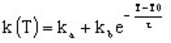# Thermal conductivity, exponential function of T

## Presentation

This model defines a conductivity k as an exponential function of the temperature for an isotropic or anisotropic material.

## Mathematical model

The thermal conductivity is an exponential function of the temperature.

The corresponding mathematical formula is written:where:

• T0 is the reference temperature degree
• ka + kb is the conductivity of a material at T0 (W/m/degree)
• ka is a constant quantity (W/m/degree)
• τ is a temperature constant (positive or negative) (degree)

The shape of k(T) curve is presented in the figure below.## Anisotropic material

For an anisotropic material, this linear model consists of a group of three exponentials.